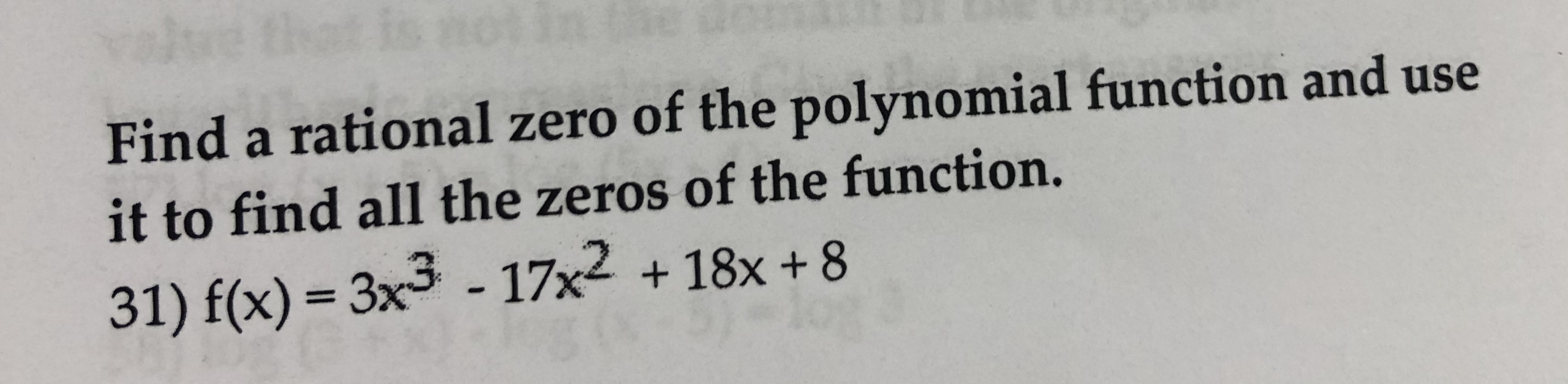Find a rational zero of the polynomial function and useit to find all the zeros of the function.31) f(x)-3x3 - 17x2 + 18x +8

Questionhelp_outlineImage TranscriptioncloseFind a rational zero of the polynomial function and use it to find all the zeros of the function. 31) f(x)-3x3 - 17x2 + 18x +8 fullscreen
Step 1

To solve the root of the  provided function  f(x)=3x3-17x2+18x+8.  Now, First use the rational theorem

a0=8, an=3

The dividers of a0 : 1,2,4,8

The dividers of a: 1,3

Therefore, check the following rational numbers: ±1,2,4,8/1,3

2/1 is the root of the expression, so factor out x-2.

Step 2

Divide the given equation by (x-2).

Step 3

Again, Divide -11x2+18x+8 by (x-...

Want to see the full answer?

See Solution

Want to see this answer and more?

Our solutions are written by experts, many with advanced degrees, and available 24/7

See Solution
Tagged in

Polynomials